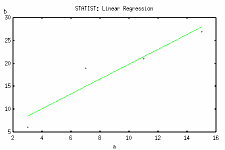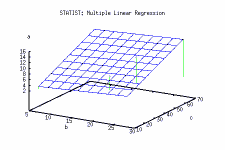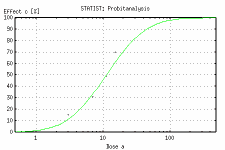# statist

statist \$B\$O!"%3%s%=!<%k%Y!<%9\$N%a%K%e!<7A<0\$K\$h\$kE}7W=hM}%W%m%0%i%`\$G\$9!#(B gnuplot \$B\$r;H\$C\$F%0%i%UI=<(\$,\$G\$-\$^\$9!#(B

\$B:n Bernhard Reiter \$B\$5\$s(B
\$B%[!<%`%Z!<%8(B: http://www.usf.uni-osnabrueck.de/~breiter/tools/statist/
(\$B%I%\$%D8l\$G\$9\$,!"(B \$B1Q8l\$N%Z!<%8(B\$B\$b\$"\$j\$^\$9(B)
\$B%P!<%8%g%s(B: 1.0.1 (2001/11/15)
\$B%i%\$%;%s%9(B: GPL

\$B%I%-%e%a%s%H\$O%I%\$%D8l\$N\$_\$G\$9\$,!"%W%m%0%i%`\$O%S%k%I;~\$K(B \$B1Q8l(B/\$B%I%\$%D8l\$,A*Br\$G\$-\$k\$h\$&\$K\$J\$C\$F\$\$\$^\$9!#(B \$B\$3\$3\$G\$4>R2p\$9\$k\$N\$O1Q8l\$G%S%k%I\$7\$?\$b\$N\$G\$9!#(B

\$B?7\$7\$\$%[!<%`%Z!<%8(B http://statist.wald.intevation.org/ \$B\$G\$O(B ver. 1.4.0 \$B\$,8x3+\$5\$l\$F\$\$\$^\$9!#(B

## \$B;H\$\$J}(B

statist \$B\$O%a%K%e! \$BNc\$(\$P!"(B

``` 3  6 15
7 19 31
11 21 49
15 27 70
```

\$B\$3\$s\$J\$U\$&\$K%G!<%?\$r5-=R\$7\$?%U%!%\$%k(B `test.dat` \$B\$rMQ0U\$7\$F!"(B

```\$ statist test.dat

STATIST --- Version 1.0.1--- (C) Dirk Melcher 1997-1999
STATIST comes with ABSOLUTELY NO WARRANTY; it is Free Software under GPL.
Read the file COPYING for details.
Hint: You can often answer "all" for the number of columns.

Column a: 4
Column b: 4
Column c: 4
--- Please, continue with <RETURN> ... ---

0 = Quit
1 = Data management
2 = Regressions and correlations
3 = Tests
4 = Miscellaneous
5 = Data manipulation

================================================

TESTS:

1 = t-test for comparison of two means of two sets
2 = t-test for comparison of pairwise ascertained data sets
3 = Test of normal distribution (KS-Lillefors-Test)
4 = Chi^2-fourfould-test
5 = Chi^2 two-items-test
6 = u-test (Test of independence of two data sets)
7 = H-test (Kruskal-Wallis) for k independent data sets
8 = Wilcoxon-Rank-test for pairwise ascertained data sets
9 = Chi^2-test of equal frequency
10 = Chi^2-test of correspondence between measured and theoretical frequency

```

\$B\$3\$s\$J46\$8\$G\$9!#(B

\$B%W%m%C%H\$NNc\$r\$\$\$/\$D\$+<(\$7\$^\$9!#(Bstatist -h \$B\$G5/F0\$9\$k\$H;HMQK!\$rI=<(\$7\$^\$9!#(B

```\$ statist -h

STATIST --- Version 1.0.1--- (C) Dirk Melcher 1997-1999
STATIST comes with ABSOLUTELY NO WARRANTY; it is Free Software under GPL.
Read the file COPYING for details.
Hint: You can often answer "all" for the number of columns.

Usage: statist <Options> data file
Options:
-h, -help, -? : print this help message and exit
-silent       : don't print menu etc. (for batch/script usage)
-log          : write results to log file `statist.log'
-nofile       : don't read a data file when starting the program
-nobell       : no beep at errors and warnings
-thist        : histogram as text graphic instead of gnuplot-graphic
-noplot       : no gnuplot-graphic
-delrow   : skip lines with missing values
--bernhard    : special output changes from Bernhard, i.e.:
- table output at miscelaneous/standard deviation
- if -noplot defined no text histogram at
miscelaneous/standard deviation

Missing values must be indecated by 'M'
```

\$B%G!<%?7gB;\$O(B M \$B\$GI=\$;\$^\$9\$,!"C1=c\$KL5;k\$5\$l\$F\$=\$N9T0J9_\$N(B \$B%+%i%`4V\$N%G!<%?\$NBP1~\$,\$:\$l\$F\$7\$^\$\$\$^\$9\$N\$GCm0U\$7\$F\$/\$@\$5\$\$!#(B

PostScript \$B7A<0\$N(B \$B%I%\$%D8l\$N%^%K%e%"%k\$O(B `/usr/share/doc/statist-1.0.1/doc/stat_man.ps` \$B\$G\$9!#(B

## \$B%\$%s%9%H!<%k(B

LinuxMLD 5,6,7 \$BMQ\$N(B RPM statist-1.0.1-1_mlb1.i386.rpm (179,900 bytes) \$B\$r%\$%s%9%H!<%k\$7\$^\$9!#(B
rpm \$B%3%^%s%I\$G%\$%s%9%H!<%k\$9\$k\$K\$O!"%9!<%Q!<%f!<%6\$K\$J\$C\$F(B

```# rpm -i statist-1.0.1-1_mlb1.i386.rpm
```

\$B\$H\$7\$^\$9!#(B
MLD 5,6 \$B\$G\$O(B Gnome \$B\$N(B GUI \$B\$G%\$%s%9%H!<%k(B\$B\$9\$k\$3\$H\$b\$G\$-\$^\$9!#(B

## \$B;29M(B

\$B4XO"%"%W%j%1!<%7%g%s(B

• StatistX -- graphical front end for statist

\$B\$=\$NB>\$NE}7W4X78\$N%"%W%j%1!<%7%g%s\$K4X\$7\$F\$O!"(B XLISP-STAT\$B!"(B R\$B!"(B pasu \$B\$N%Z!<%8\$r;2>H\$7\$F\$/\$@\$5\$\$!#(B

[2002/01/14 \$B:n@.(B] [2003/10/30 \$B99?7(B]

\$B\$3\$N%Z!<%8\$K4X\$9\$k8f0U8+!"8fMWK>\$r(B science@mlb.co.jp \$B\$^\$G\$*4s\$;2<\$5\$\$(B